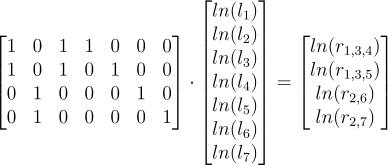# Subtract Matrices Calculator

Instructions: Use our step-by-step calculator of the subtraction of two matrices, by providing your two matrices of the same size .

Modify, if needed, the size of the matrices by indicating the number of rows and the number of columns. Once you have the correct dimensions you want, you input the matrices (by typing the numbers and moving around the matrix using "TAB")

Number of Rows =    Number of Cols =

$$A$$ = \begin{bmatrix} & \\ & \end{bmatrix}

$$B$$ = \begin{bmatrix} & \\ & \end{bmatrix}

## More about Subtraction of Matrices Calculator

As you probably know by now, matrices is are very common object that has a recurrent appearance in Algebra, in the most diverse contexts.

Matrices have very interesting properties, such invertibility, which is easily assessed by computing the determinant of the matrix. Whether a matrix is invertible or not will depend to whether we can "divide" by that matrix.### How do you subtract matrices?

Matrices, when they have the correct dimensions, can be operated in different ways. For example, matrices can be subtracted, as long as the matrices you are subtracting have the same dimensions.

The action of the subtraction itself is fairly simple: you just simply go subtracting ALL the matrices components, one by one. Below you have a handy list of steps to follow, when you want to subtract matrices:

Step 1: You need to ensure that the matrices you are operating (subtracting in this case) have the same dimension. In order to actually proceed with the subtraction, you want both matrices to have the same number of columns and the same number of rows.

Step 2: If, and only if the matrices have the same size, then you can construct the resulting subtraction matrix by subtracting component by component of the two matrices you are subtracting.

### Can I, for example, subtract a 3x3 matrix from 3x4 matrix?

The answer is NO. You want them to have the same dimension. You cannot subtract a 3x3 matrix from 3x4 matrix, but for example, you can subtract a 3x3 matrix from 3x3 matrix, OR you can also subtract a 3x4 matrix from 3x4 matrix

Of course! As long as the matrices have the same dimensions, you can also add two matrices by adding component by component.

Not only adding and subtracting, you can also compute the multiplication of two matrices, when the number of columns of the first matrix is the same as the number of rows of the second matrix.